# 结对第二次—文献摘要热词统计及进阶需求

Github仓库地址 github

## 1.Github的代码签入记录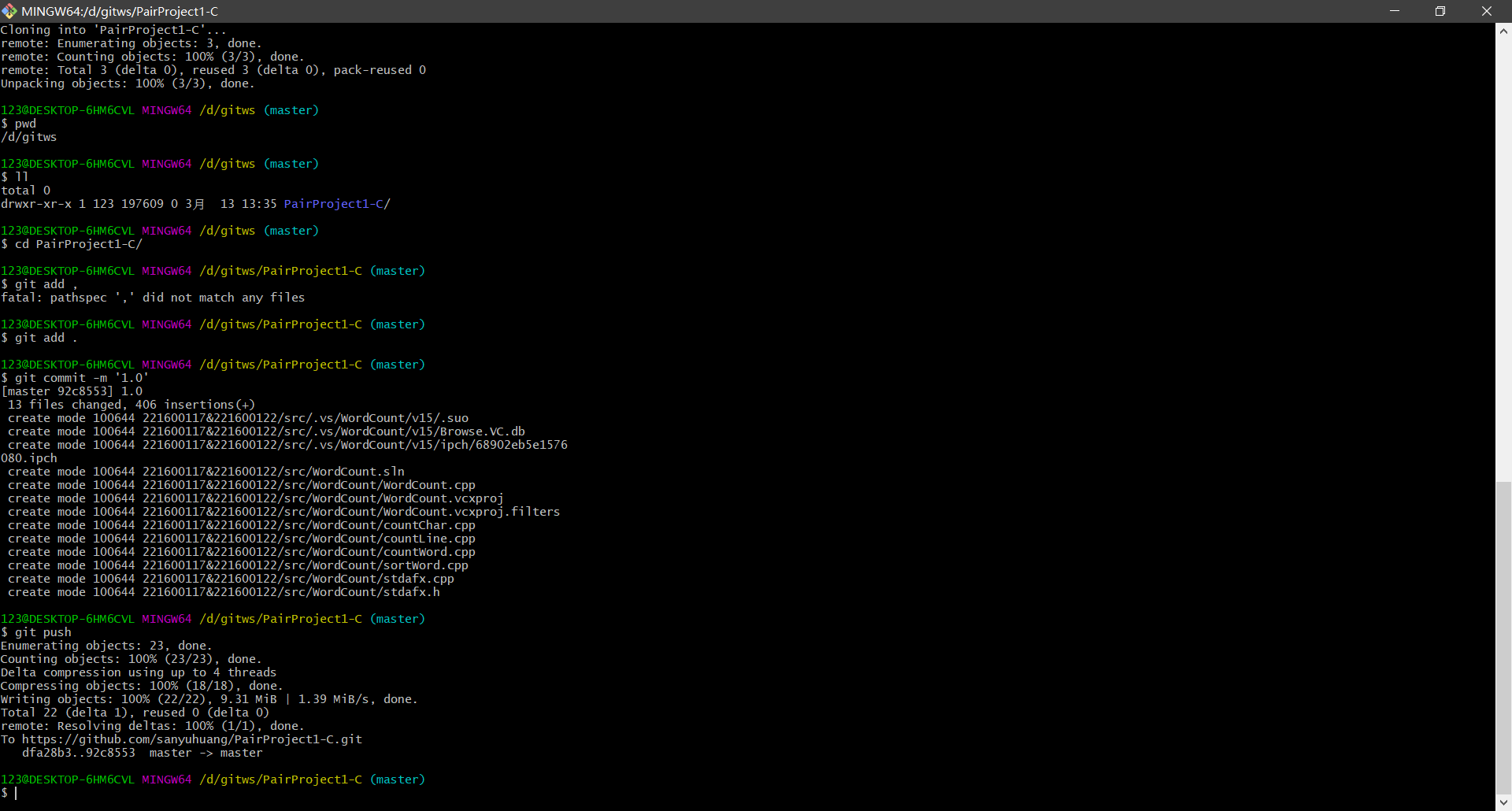## 2.具体分工

​ 我们两个在这次作业中一起实现了基础部分的实现，每个人写了两个功能，队友主要负责基础部分代码的编写和单元测试部分，还有git的使用方法的学习，基础部分博客编写。我主要负责进阶部分的代码编写与相关部分的博客。

## 3.PSP表格

PSP2.1 Personal Software Process Stages 预估耗时（分钟） 实际耗时（分钟）
Planning 计划
• Estimate • 估计这个任务需要多少时间 480
Development 开发
• Analysis • 需求分析 (包括学习新技术) 120 150
• Design Spec • 生成设计文档 15 20
• Design Review • 设计复审 10 5
• Coding Standard • 代码规范 (为目前的开发制定合适的规范) 5 1
• Design • 具体设计 20 10
• Coding • 具体编码 180 240
• Code Review • 代码复审 30 10
• Test • 测试（自我测试，修改代码，提交修改） 60 60
Reporting 报告
• Test Report • 测试报告 20 15
• Size Measurement • 计算工作量 10 5
• Postmortem & Process Improvement Plan • 事后总结, 并提出过程改进计划 10 5

## 4.解题思路

​ 首先拿到题目后，看完了整个题目后，感觉基础部分实现起来并不是特别困难，我们就准备第二天开始着手解决基础部分的代码。我先学习了git的使用方法，通过百度找一些别人写的博客了解了git的基本使用方法。这部分花了一上午的时间。

​ 在接下来就是对于代码实现题目的思考。看到题目要求对字符进行处理统计，就决定使用C++来实现，因为C++对字符的操作更加简单，也不需要特别复杂的操作。

​ 找资料的过程就是通过在网上搜索一些c++中对字符处理的函数方法，距离上次使用C++已经有一段时间了，这次正好复习了一下C++。

​ 看到需求中有要求对代码进行单元测试，还有进行性能分析。这些以前都没有做过，所以学起来也花了一部分时间。网上对于这两部分的讲解资料并不是特别多。

## 5.设计实现过程

1. 命令行测试程序使用
2. 在单元测试框架下使用
3. 与数据可视化部分结合使用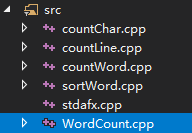• 测试字符数是否正确
• 统计单词数是否正确
• 测试行数是否正确
• 测试如果是不存在的文件
• 测试如果是空文件

### 算法设计过程

#### 需求一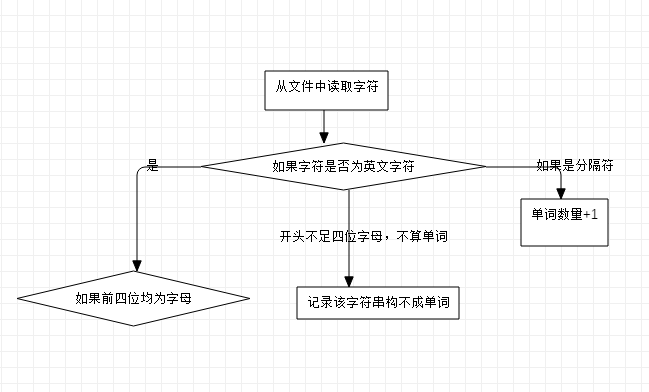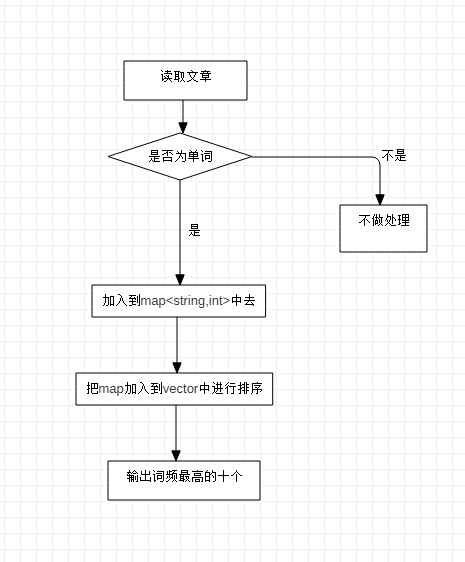while((ch = fgetc(file)) != EOF)
{
if (ch >= 'a' && ch <= 'z' || ch >= 'A' && ch <= 'Z')
{
if (f >= 0)//单词前四位是字母
f++;
else//单词前字母不足四位，后面字母不记录
f = -1;
}
else if (ch >= '0' && ch <= '9' && f < 4)//开头不足四位字母，不算单词
{
f = -1;
}
else//读到分隔符
{
if (f >= 4)
cnt++;
f = 0;
}
}
//若最后一个单词符合条件且到文件末尾
if (f >= 4)
cnt++;


while ((ch = fgetc(file)) != EOF)
{
//遇到换行符
if (ch == '\n')
{
//本行内容为非空字符
if (f > 0)
cnt++;
f = 0;
}
//遇到非空字符
else if(ch != ' ' && ch != '\t')
f++;
}
//最后一行不含回车符，特殊判断
if (f > 0)
cnt++;


while(fgetc(file) != EOF)
{
cnt++;
}


while ((ch = fgetc(file)) != EOF)
{
if (ch >= 'a' && ch <= 'z' || ch >= 'A' && ch <= 'Z')
{
if (ch >= 'A' && ch <= 'Z')
{
ch += ('a' - 'A');
}
if (f >= 0)//单词前四位是字母
{
f++;
temp += ch;
}
else//单词前字母不足四位，后面字母不记录
f = -1;
}
else if (ch >= '0' && ch <= '9')//开头不足四位字母，不算单词
{
if (f < 4)
f = -1;
else
temp += ch;
}
else//读到分隔符
{
if (f >= 4)
{
cnt++;
wmap[temp]++;
}
f = 0;
temp = "";
}
}
if (f >= 4)
{
cnt++;
wmap[temp]++;
}
MapSortOfValue(vec, wmap);


### 需求二

​ 爬虫：使用javajsoup库爬取HTML文档，然后生成DOM文档。在DOM树上，寻找到每一篇论文中的href链接发送请求，对于收到的文本中，解析出需要的Title和Abstract内容。

​ 统计词组方面，主要难点是解决规定的词组长度截取以及注意不能跨越两个栏目，解决思路是：利用一个向量来模拟队列。规定队列长度为词组长度，新单词进入加入队尾，超过长度则抛出队头，当队伍长度等于词组长度时，队列就是保留一个合法词组。当遇到Title与Abstract时，队列清空，就可以做到不跨越两个栏目。剩下排序就和需求一相同。核心部分流程图如下：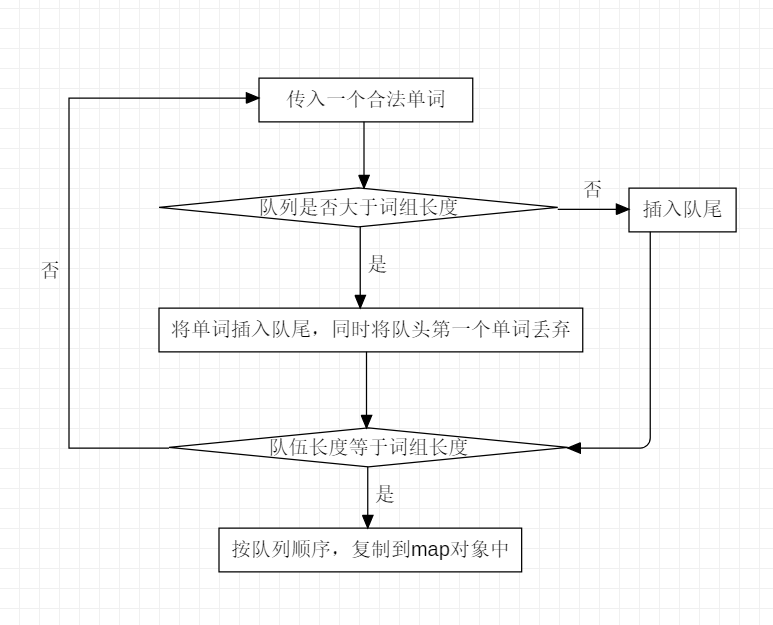//......
while ((ch = fgetc(file)) != EOF)
{
if (ch >= 'a' && ch <= 'z' || ch >= 'A' && ch <= 'Z')
{
if (ch >= 'A' && ch <= 'Z')
{
ch += ('a' - 'A');
}
if (f >= 0)//单词前四位是字母
{
f++;
temp += ch;
}
else//单词前字母不足四位，后面字母不记录
f = -1;
}
else if (ch >= '0' && ch <= '9')//开头不足四位字母，不算单词
{
if (f < 4)
f = -1;
else
temp += ch;
}
else//读到分隔符
{
//当遇上title或者abstract时，模拟队列清空
if (temp == "title" && temp == "abstract")
que.clear();

if (f >= 4 && temp != "title" && temp != "abstract")
{
que.push_back(temp);
//维持词组的规定长度
if (que.size() > Len)
que.erase(que.begin());
//符合长度，加入词组map中
if (que.size() == Len)
{
tt = "";
k = 0;
for (vt = que.begin(); vt != que.end(); vt++)
{
k++;
tt += *vt;
if (k < Len)
tt += " ";
}
wmap[tt]++;
}
}
f = 0;
temp = "";
}
}
//......


## 6.需要改进的地方

​ 在计算词频出现的时候，需要判断是否为单词，这部分和在统计单词数量的时候的代码有部分重合了，也就是说需要重复的计算相同的部分，会花掉一部分时间，这部分代码的设计存在着问题。

## 7.单元测试部分

TEST_METHOD(TestMethod1)//测试字符数是否正确
{
FILE *file;
char* fn = "input1.txt";
fopen_s(&file, fn, "r");
int count = 102;
Assert::AreEqual(countChar(fn), count);
}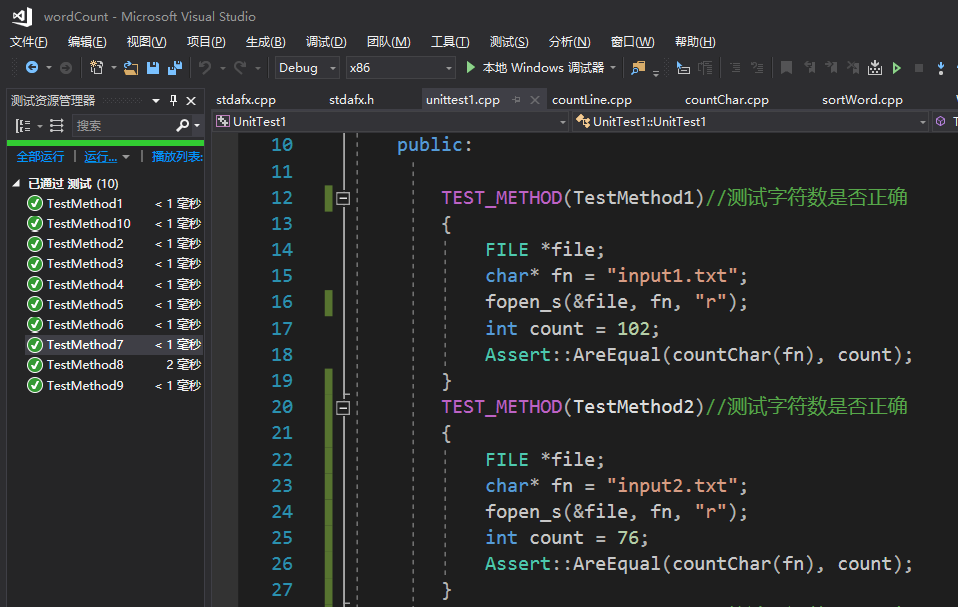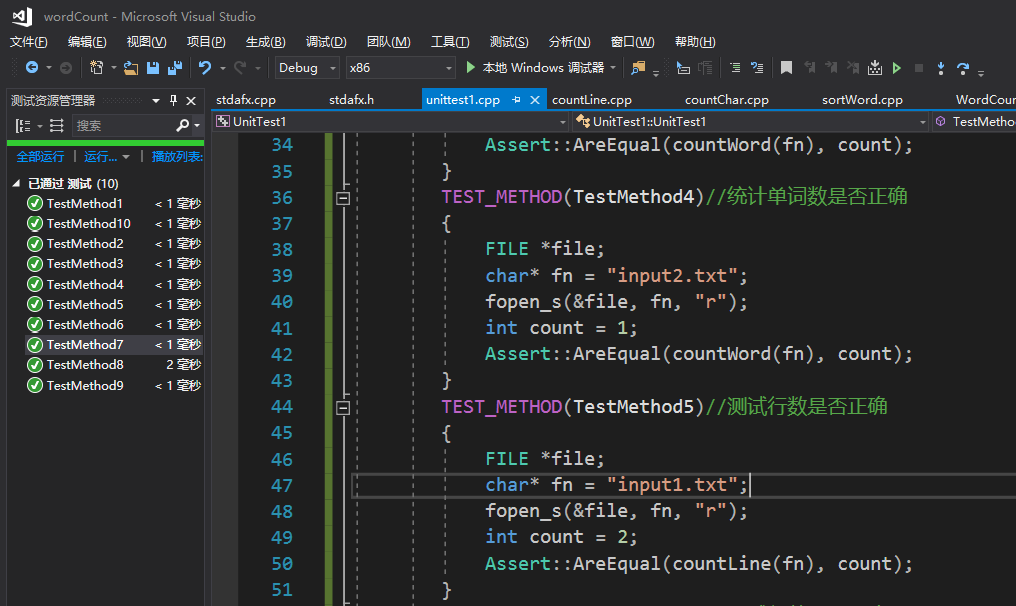## 8.性能分析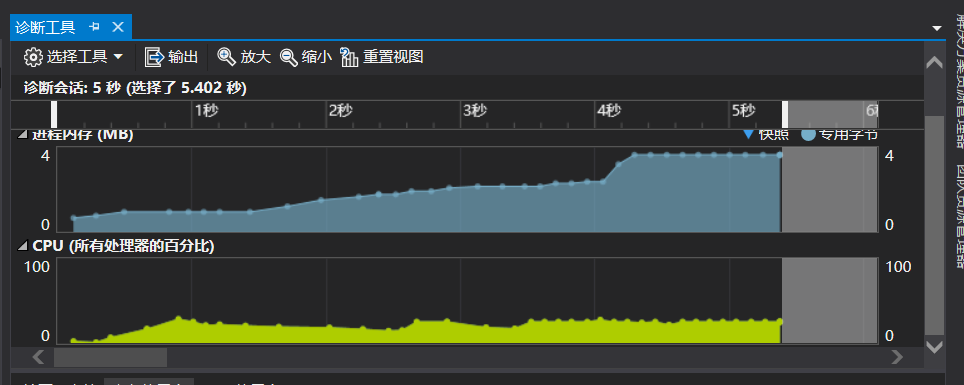## 9.遇到的困难

​ 关于单元测试和性能分析，是以前从来没有接触过的，只能在网上查找资料来学习。但是相关的资料非常的不好找。所以资料就花掉了很多时间。还有git的使用，不过还好有Linux使用的基础，学起来也还相对轻松。

​ 剩下的困难就是在代码方面的困难了。理解题目需求花了很多时间，经常写着写着发现好像不符合题目的要求，然后就又重新改。

​ 解决办法就是一定要先理解了需求，再开始着手。不然后期会很麻烦，前面努力都白费了。

## 10.评价队友

posted @ 2019-03-15 20:48  弄影丶  阅读(...)  评论(...编辑  收藏